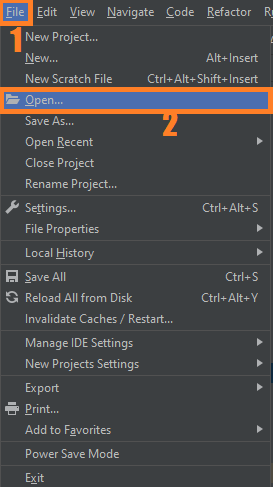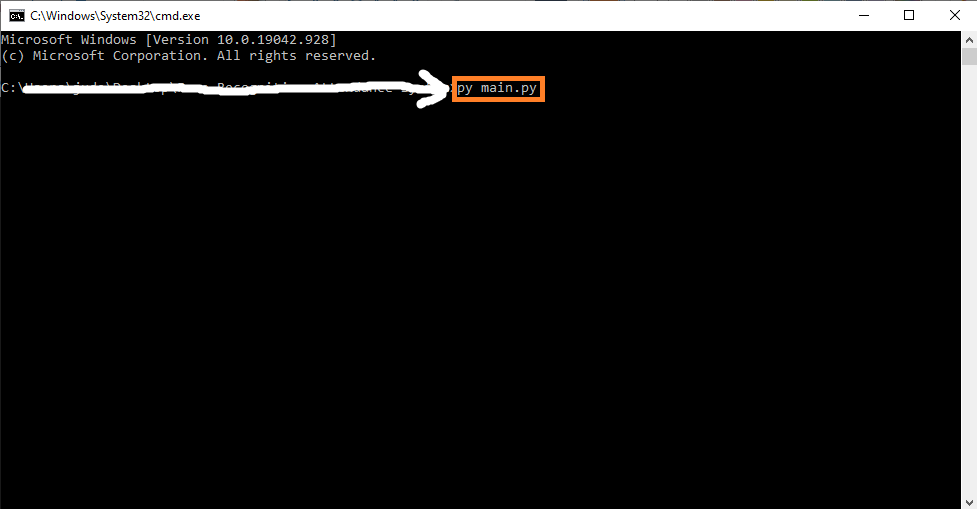The Sudoku Game Python Code is one of all time’s most famous puzzle games. The main objective of the project is to fill a 4 range chart (i.e. 4×4 grid) with numbers so that every segment of the row, column, and 2 range contains all the numbers between 1 and 4.

A Sudoku Game Code in Python is also a great brain game as a logic puzzle. Also, this game helps the players to improve their brain capacity. Some of the AI algorithms also has implemented for solving Sudoku game.

## How To Play Sudoku Game In Python?

The goal of the Sudoku is to fill a 4 range chart (i.e. 4×4 grid) with numbers so that every segment of the row, column, and 2 range contains all the numbers between 1 and 4.

4×4 grid will have some of the squares filled in at the beginning of the game. The player’s work is to fill in the missing digits and complete the grid using logic. The moves will be incorrect if:

• Any row contains more than one of the same number from 1 to 4
• Whichever column contains more than one of the same number from 1 to 4.
• Any 2×2 grid contains more than one of the same number from 1 to 4.

To start executing a Sudoku Game Python Code, make sure that you have installed Python 3.9 and PyCharm in your computer.

## Sudoku Game Python Code : Steps on how to run the project

Time needed: 5 minutes.

These are the steps on how to run Sudoku Game Python Code

First, download the given source code below and unzip the source code.• Step 2: Import the project to your PyCharm IDE.• Step 3: Run the project.

last, run the project with the command “py main.py”## Install Libraries

``````import turtle
import random``````

## Complete Source Code

``````import turtle
import random

# --------------- Made this function to set our coordinates and environment whenever we play ------------- #
def setWorld():
turtle.setworldcoordinates(-100, -100, 100, 100)
turtle.hideturtle()
turtle.pensize(10)
turtle.speed(0)
turtle.pu()
turtle.goto(-75, -75)

# ------------ A function to draw the outer large square ------------- #
def drawGrid():
for i in range(2):
turtle.fd(150)
turtle.lt(90)
turtle.fd(150)
turtle.lt(90)

# ------------ A function to draw squares within the grid ---------#
def drawSquare():
for i in range(2):
turtle.fd(37.5)
turtle.lt(90)
turtle.fd(37.5)
turtle.lt(90)

# ------------- A function to keep drawing squares and moving forward until it reaches a specific x-coordinate --------#
def move_turtle():
turtle.pd()
while not (turtle.xcor() >= 75):
drawSquare()
turtle.setx(turtle.xcor() + 37.5)

# ---------------- 'move_turtle()' draws squares in one row.  ---------------------- #
def make_box():
row_boxes = 0
turtle.sety(-37.5)
while row_boxes != 4:
move_turtle()
turtle.setx(-75)  # Start making boxes from the left for each row
turtle.sety(
turtle.ycor() + 37.5)  # Once boxes are filled in one row, move y coordinate up. E.g (Makes boxes along row 'D', now  move up
row_boxes += 1  # Do this until boxes are made in all four rows.
turtle.pu()

# ------------- A function to label the grid -------------- #
def labelGrid():
rows = ['A', 'B', 'C', 'D']
cols = ['1', '2', '3', '4']

for row_name in rows:
turtle.write(row_name, move=False, align='left', font=('Georgia', 40, 'normal'))
turtle.sety(turtle.ycor() - 40)

turtle.goto(-65, 75)
for col_name in cols:
turtle.write(col_name, move=False, align='left', font=('Georgia', 40, 'normal'))
turtle.setx(turtle.xcor() + 40)

# --------------- A function that uses the turtle class as an eraser ---------- #
def eraseEntry(puzzle):
'''
Takes in the puzzle
Asks the user if they want to erase the entry before hand
'''
eraser = turtle.Turtle()
eraser.hideturtle()
eraser.speed(0)
eraser.pu()

ask_user = turtle.textinput('', 'Do you want to erase the previous entry? (y/n)')
if ask_user in ['y', 'YES', 'yes']:
eraser.goto(turtle.xcor() + 5,
turtle.ycor())  # I had to adjust where the eraser goes accordingly. By trial and error I just had to move it a bit
eraser.pd()  # to the right.
eraser.color('white', 'white')
eraser.begin_fill()
eraser.circle(10)  # Using a white circle to be my eraser
eraser.end_fill()

def take_input(puzzle):
grid_rows = ['A', 'B', 'C', 'D']
grid_cols = ['1', '2', '3', '4']

col_increment_size = [-60, -30, 10, 50]
row_increment_size = [50, 10, -30, -60]

user_input = turtle.textinput('', 'Enter Row and Column (e.g B4)')

x_cor = 0
y_cor = 0

for r in range(0, len(grid_rows)):
if grid_rows[r] == user_input:  # First 'element' of the user's input will be the row. E.g B1
y_cor = row_increment_size[r]

for c in range(0, len(grid_cols)):
if grid_cols[c] == user_input:  # Second 'element' of the user's input will be the column.
x_cor = col_increment_size[c]

turtle.goto(x_cor, y_cor)

# Appending the puzzle (which is a nested list)

get_input = turtle.textinput('', 'Enter a number and make your move!')

for i in range(0, len(grid_rows)):
if user_input == grid_rows[i]:
for j in range(0, len(grid_cols)):
if user_input == grid_cols[j]:
if puzzle[i][j] != 0:  # Call the eraseEntry function and ask
eraseEntry(puzzle)  # Ask the user if they want to erase the entry where there isn't a zero
puzzle[i][j] = int(get_input)
make_move = turtle.write(get_input, move=False, align='left', font=('Arial', 35, 'normal'))

return puzzle

def populatePuzzle(puzzle):
'''
Takes in a puzzle (which is a nested list)
Returns the grid with the initial numbers inside
'''

col_increment_size = [-60, -30, 10, 50]  # Starts at A1 (-60,50), A2 (-30,50), A3 (10,50) .... D4 (50,-60)
row_increment_size = [50, 10, -30, -60]

for row in range(0, len(puzzle)):  # Enter the row
for col in range(0, len(puzzle[row])):  # Enter the the row and which column the element belongs to
if puzzle[row][col] != 0:  # If the initial base is not 0, populate the grid
turtle.goto(col_increment_size[col], row_increment_size[row])
turtle.write(puzzle[row][col], move=False, align='left', font=('Arial', 35, 'normal'))

def row_check(puzzle):
'''
Assumes that puzzle is a nested list
Returns True if every element in the row is unique
'''

check = 0
for row in range(0, len(puzzle)):
if len(puzzle[row]) == len(set(puzzle[row])) and 0 not in puzzle[
row]:  # Using the notion of sets, we look at the length of each row
check += 1  # Check keeps track of how many rows (same for columns and box)
# are unique. If all 4 are unique, return True.
if check == 4:
return True

else:
return 'Keep trying!'

def col_check(puzzle):
'''
Assumes that puzzle is a nested list
Returns True if every element in the column is unique
'''

col_1 = [s for s in puzzle]  # Turns each 'row' of the puzzle into a column. Kind of how you invert a matrice
col_2 = [s for s in puzzle]  # so for example. col_1 = [A1, B1, C1, D1], col_2 = [A2, B2, C2, D2] and so on.
col_3 = [s for s in puzzle]  # After the transformation is done, it applies the checker. The checker looks
col_4 = [s for s in puzzle]  # at the length of each column. If every number is unique len(set(col_1)) = 4 and
all_col = [col_1, col_2, col_3, col_4]  # if that matches with our actual column return True.

check = 0
for col in range(0, len(all_col)):
if len(all_col[col]) == len(set(puzzle[col])) and 0 not in all_col:
check += 1

if check == 4:  # 4 because 4 columns
return True

else:
return 'Keep trying!'

def box_check(puzzle):
'''
Assumes that puzzle is a nested list
Rearragnes the puzzle so that each 'row' is a box.
'''

box_1 = [s[0:2] for s in
puzzle[0:2]]  # Transforming each row of the puzzle so that all elements are rearranged as boxes
box_2 = [s[2:] for s in puzzle[0:2]]  # so for example, box_1 = [A1,A2,B1,B2], box_2 = [A3,A4,B3,B4] and so on
box_3 = [s[0:2] for s in puzzle[2:]]
box_4 = [s[2:] for s in puzzle[2:]]

all_box = [box_1, box_2, box_3, box_4]

check = 0

for box in all_box:
if len(box + box) == len(set(box + box)) and 0 not in box + box:
check += 1

if check == 4:
return True

else:
return 'Keep trying!'

# ----------------- A function to see if the board is filled ----------------- #
def isboardfilled(puzzle):
'''
Assumes puzzle is a nested list
Returns True if the number of 'non_zero' elements match the puzzle
'''

non_zero = []
for row in range(len(puzzle)):
for el in range(len(puzzle[row])):
if puzzle[row][el] != 0:
non_zero.append(puzzle[row][el])
if len(non_zero) == len(puzzle[row]) * len(
puzzle):  # len(puzzle[row]) -> Number of elements in each row
return True  # len(puzzle) -> Number of rows.
# Therefore total numbers = len(puzzle[row])* len(puzzle)

def replay():
return turtle.textinput('', "Do you want to play again? (y/n)") in ['yes', 'y', "YES"]

# ------------------- Pick one of these random puzzles to start the game --------------------------- #

puzzle_1 = [[3, 4, 1, 2], [0, 0, 0, 0], [0, 0, 0, 0],
[4, 2, 3, 1]]  # Solution: [[3,4,1,2],[2,1,4,3],[1,3,2,4],[4,2,3,1]]
puzzle_2 = [[4, 0, 0, 1], [0, 1, 3, 0], [0, 4, 1, 0],
[1, 0, 0, 3]]  # Solution: [[4,3,2,1],[2,1,3,4],[3,4,1,2],[1,2,4,3]]
puzzle_3 = [[0, 0, 0, 0], [2, 3, 4, 1], [3, 4, 1, 2],
[0, 0, 0, 0]]  # Solution: [[4,1,2,3],[2,3,4,1],[3,4,1,2],[1,2,3,4]]
puzzle_4 = [[0, 2, 4, 0], [1, 0, 0, 3], [4, 0, 0, 2],
[0, 1, 3, 0]]  # Solution: [[3,2,4,1],[1,4,2,3],[4,3,1,2],[2,1,3,4]]
puzzle_5 = [[0, 4, 2, 0], [2, 0, 0, 0], [0, 0, 0, 3],
[0, 3, 1, 0]]  # Solution: [[3,4,2,1],[2,1,3,4],[1,2,4,3],[4,3,1,2]]

all_puzzles = [puzzle_1, puzzle_2, puzzle_3, puzzle_4, puzzle_5]

# ---------------------------------------------   Start the game   ---------------------------------------------------------- #

while True:
turtle.reset()  # Start with a clean canvas in case user wants to replay
setWorld()
game_won = False
turtle.pd()
drawGrid()

# --------- Draw the lines in bold, across and down -------- #
turtle.pu()
turtle.goto(-75, 0)
turtle.pd()
turtle.fd(150)
turtle.pu()
turtle.goto(0, 75)
turtle.lt(270)
turtle.pd()
turtle.fd(150)
turtle.pu()

# -------- Make the turtle go to the left ------------- #
turtle.goto(-75, -75)

turtle.pensize(2)
make_box()
turtle.goto(-95, 45)  # Start by going to the left top of the grid (Labeling starts with 'A', 'B'... etc)
labelGrid()
game_puzzle = all_puzzles[random.randint(0, 4)]  # Pick a random puzzle to start the game
populatePuzzle(game_puzzle)

while game_won != True:
take_input(game_puzzle)

if isboardfilled(game_puzzle):
confirm_sol = turtle.textinput('', 'Are you sure you want to submit this solution? (y/n)')
if confirm_sol in ['yes', 'y', 'YES']:
if row_check(game_puzzle) == col_check(game_puzzle) == box_check(game_puzzle) == True:
turtle.textinput('', 'Congratulations you have won! (Press any key to continue)')
game_won = True
else:
turtle.textinput('', "Your solution is incorrect. Keep trying! (Press any key to continue)")

if not replay():
break

turtle.done()``````